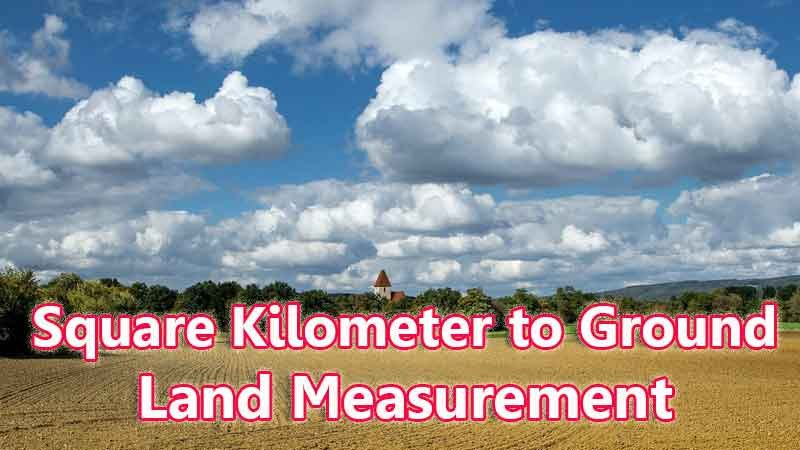# Square Kilometer To Ground Converter

A Square Kilometer unit is a vital thing to measure the land area and this came from the SI unit measurement. This Km square is notably used to find the area of land occupied by a farm, city, and more. It just mentions the area inside a square and that square side is equal to One Kilometer or 1000 meters. And one interesting thing to know here is “ India’s total area would be mentioned in Square Kilometer and that is 3.287 million kilometer square”.

Interestingly, we are given this Square Kilometer to Ground unit converter to find this large standard unit to Southern traditional unit “Ground” count.

Input Here

square kilometer

Output

ground

1 square kilometer = 4483.333333333333 ground
Rounded Value Is (1 square kilometer = 33 ground)

## Measurement

square kilometer means - a unit of land measurement. This tool provides great calculation over square kilometer to ground, 1 square kilometer to ground, 1 square kilometer in ground, 1 square kilometer equal to and etc. To convert square kilometer to ground, then multiply the unit value by 4483.333333333333. Then, 1 square kilometer * 4483.333333333333 = 4483.333333333333 ground. Hence, 1 square kilometer is equal to 4483.333333333333 ground.

A Ground is the most popular unit in the South and Central parts of India. This measurement unit is also one of the famous among these units Cent, Square feet, ankanam, and guntha in the rustic side of India. Ground units are mainly used for land real estate and construction work area measurement purposes. Moreover, it traditionally starts before the 20 th century, and half a unit of ground area was used to build small individual homes in the States of India. So, use this Convertor to find the Square kilometer to Ground unit by your own value.

ground also comes under this land measurement unit and we are comparing values between square kilometer traditional unit with other side land measurement unit.

## Formula For square kilometer to ground Conversion(square kilometer to ground formula)

The base formula for this square kilometer to ground converter is

ground = square kilometer * 4483.333333333333

## How many ground in a square kilometer?

Step 1: To Convert 1 square kilometer to ground

Step 2: Applying formula ground = square kilometer * 4483.333333333333, (i.e) multiply the unit value by 4483.333333333333.

Step 3: Then, ground = 1 * 4483.333333333333 = 4483.333333333333.

Step 4: Hence, 1 square kilometer is equal to 4483.333333333333 ground.Some square kilometer to ground Conversion Chart for your reference:

 1 square kilometer = 4483.333333333333 ground 2 square kilometer = 8966.666666666666 ground 3 square kilometer = 13450 ground 4 square kilometer = 17933.333333333332 ground 5 square kilometer = 22416.666666666668 ground 6 square kilometer = 26900 ground 7 square kilometer = 31383.333333333332 ground 8 square kilometer = 35866.666666666664 ground 9 square kilometer = 40350 ground 10 square kilometer = 44833.333333333336 ground

The square kilometer to ground conversion chart is above listed for your reference. This chart, however, represents the simple math calculation involved in the square kilometer to ground convertion online.

For Example: How much is 1 square kilometer to ground

Solution:

= (square kilometer * 4483.333333333333)

= (1 x 4483.333333333333)

= 4483.333333333333 ground

For Example: How much is 120 square kilometer in ground

Solution:

= (square kilometer * 4483.333333333333)

= (120 x 4483.333333333333)

= 538000 ground

This formula provides an instant answer for all your questions / People also search:

• 1 square kilometer to ground
• 1 square kilometer is equal to how many ground
• Formula to convert square kilometer to ground online
• How to convert square kilometer to ground?
• How much ground is equal to a one square kilometer?
• How many ground in square kilometer?
• 1 square kilometer equal to ground